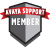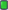Thread: Problem w/ analog card View Single Post
#1
 jpferraraMemberJoin Date: Jul 2013 Posts: 4Problem w/ analog card

I have a CS100 branch office that I put an additional analog card in & the TNs that I've configured are coming up w/ fast busy. I have enough analog licenses on the system. When I stat the card it comes up as idle.

REQ: stat 4 0 2

00 = UNIT 00 = IDLE (L500)
01 = UNIT 01 = UNEQ
02 = UNIT 02 = IDLE (L500)
03 = UNIT 03 = UNEQ
04 = UNIT 04 = UNEQ
05 = UNIT 05 = UNEQ
06 = UNIT 06 = UNEQ
07 = UNIT 07 = UNEQ
08 = UNIT 08 = UNEQ
09 = UNIT 09 = UNEQ
10 = UNIT 10 = UNEQ
11 = UNIT 11 = UNEQ
12 = UNIT 12 = UNEQ
13 = UNIT 13 = UNEQ
14 = UNIT 14 = UNEQ
15 = UNIT 15 = UNEQ

So, what am I missing?